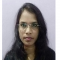## PHP – How to get the square root of an arbitrary precision number using bcsqrt() function?Urmila Samariya

Updated on 21-Aug-2021 07:39:27

In PHP, the bcsqrt() function is used to get the square root of an arbitrary precision number. It accepts the arbitrary precision number as a string and gives the square root of the number after scaling the result to the specified precision.Syntaxstring bcsqrt(\$num_string, \$scale)ParametersThe bcsqrt() function accepts two different parameters: ... Read More

## PHP – How to set or get the default scale parameter for all bc math functions using bcscale() function?Urmila Samariya

Updated on 21-Aug-2021 07:37:33

In PHP, bcscale() function is used to set the default parameter for all bc math functions. This function sets the default scale parameter for all following calls to the bc math functions that do not explicitly specify a scale parameter.Syntaxint bcscale(\$scale)ParametersThe \$bcscale() parameter accepts only a single parameter and it ... Read More

## PHP – bcpowmod() functionUrmila Samariya

Updated on 21-Aug-2021 07:35:50

In PHP, bcpowmod() function is used to raise an arbitrary precision base number to another exponent number, reduced by a specified modulus. The bcpowmod() function accepts three arbitrary precision numbers as strings and it returns the base number raised to the exponent modulo number after scaling the result to the ... Read More

## PHP – How to subtract one arbitrary precision number from another using bcsub() function?Urmila Samariya

Updated on 21-Aug-2021 07:34:30

In PHP, bcsub() math function is used to subtract one arbitrary precision number from another number. The bcsub() function takes two arbitrary precision numbers as strings and it gives the subtraction of the two numbers after scaling the result to an identified precision.Syntaxstring bcsub (\$num_str1, \$num_str2, \$scaleVal)ParametersThe bcsub() math function ... Read More

## PHP – How to compare two arbitrary precision numbers using bccomp() function?Urmila Samariya

Updated on 21-Aug-2021 07:32:02

In PHP, bccomp() function is used to compare two arbitrary numbers. The bccomp() function takes two arbitrary precision numbers as strings and gives the output as an integer after comparing the two numbers.Syntaxint bccomp(\$left_string1, \$right_string1, \$scaleval)ParametersThe bccomp() function accepts three different parameters− \$left_string1, \$right_string2 and \$scaleval.\$left_string1− It represents the left ... Read More

## PHP – How to divide two arbitrary precision numbers using bcdiv() function?Urmila Samariya

Updated on 21-Aug-2021 07:29:52

In PHP, bcdiv() math function is used to divide one arbitrary precision number from another number. The bcdiv() function takes two arbitrary precision numbers as strings and it gives the result as a division of two numbers after scaling the result to an identified precision. Or, we can say that ... Read More

## PHP – How to get the modulus of an arbitrary precision number using bcmod() function?Urmila Samariya

Updated on 21-Aug-2021 07:27:15

In PHP, bcmod() math function is used to calculate the modulus of an arbitrary precision number. The bcmod() function takes an arbitrary precision number as strings and it gives the result as a modulus of numbers after scaling the result to an identified precision. Or, we can say that it ... Read More

## PHP – How to multiply two arbitrary precision numbers using bcmul() function?Urmila Samariya

Updated on 21-Aug-2021 07:24:52

In PHP, bcmul() math function is used to multiply one arbitrary precision number with another number. The bcmul() function takes two arbitrary precision numbers as strings and it gives the result as the multiplication of two numbers after scaling the result to an identified precision.Syntaxstring bcmul( \$num_string1, \$num_string2, \$scaleVal)ParametersThe bcmul() ... Read More

## PHP – How to raise an arbitrary precision number to another using bcpow() function?Urmila Samariya

Updated on 21-Aug-2021 07:21:51

In PHP, bcpow() function is used to raise an arbitrary precision base number to another exponent number. It takes two arbitrary precision numbers as strings and gives the base number raised to the power exponent after scaling the result to the listed precision.SyntaxString bcpow(\$base, \$exponent, \$scale)ParametersThe bcpow() function in PHP takes ... Read More

## PHP – How to add two arbitrary precision numbers using bcadd() function?Urmila Samariya

Updated on 21-Aug-2021 07:20:13

In PHP, bcadd() math function is used to add two arbitrary precision numbers. The bcadd() function takes two random precision numbers as strings and it returns the addition of the two numbers after scaling the result to an identified precision.Syntaxstring bcadd ( \$num_str1, \$num_str2, \$scaleVal)ParametersThe bcadd() math function accepts three ... Read More

Previous 1 ... 5 6 7 8 9 10 11 ... 15 Next